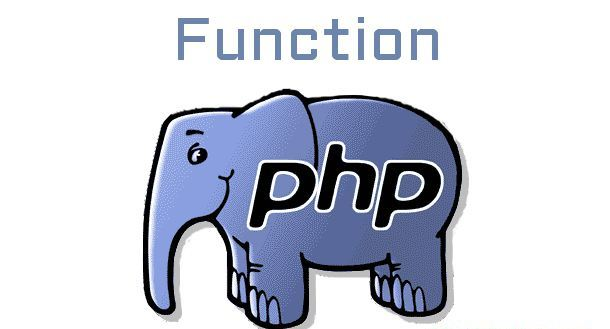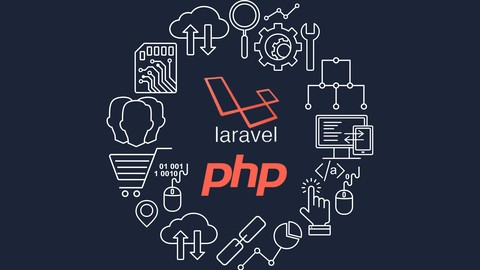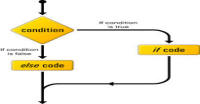# Functions in php

In a program we have very long code. This code is distributed in number of files, classes and functions. Functions are the set of statements which perform similar task. For example in authentication system, login, register and logout are the functions.

### Simple functions

The syntax of simple function is:

```<?php
function function_name()
{
//code
}

?>
```

Just like variable name, you can give any name to function (in above we have given name as function_name). The code is written inside the curly brackets. This code executes when we call this function (just like built-in functions). We call function by its name followed by brackets.

```<?php

// you can call function also here before defining

// defining function with name say_hello
function say_hello()
{
// this fuction is printing hello
echo "hello";
}

// calling the function
say_hello();

?>
```

### Function with parameters

We can send extra information (like username and password) to the functions using the arguments. For this, we have to define parameters.

NOTE: Arguments are the data which we are passing to function when we call it and parameters are the variables which we define when we write the code for function. We can assume parameters as holes and arguments as data which we are putting in those holes.

```<?php

// login function with two parameters
{
}

// calling the function

// calling the function

?>
```

NOTE:

Parameters are local variables of the function and can only be accessible within the function.

### Function with default value

We can give default values to the parameters and such parameters can be escape when we call the function.

```<?php

// login function with two parameters
function login(\$user, \$pass, \$active = "not active")
{
echo "hello, username is \$user and password is \$pass and user is \$active </br>";
}

// calling the function

// calling the function

?>
```

NOTE: If you want to skip a argument in function call then corresponding parameter must be on the last position just like in the above example. Every parameter has null default value (if you have not defined your own), so you can skip last variables any time.

### Function with variable parameters

Functions can have variable parameters. In fact we can pass any number of arguments to the function. To access these arguments we have to use following built-in functions:

1. func_get_args( ): It returns array of all arguments
2. func_num_args( ): It returns total number of arguments
3. func_get_arg(argument_number): It returns argument at position argument_number

In this example, we are returning sum of the numbers which are passing to the function as arguments and also printing those arguments.

```<?php

function sum()
{
// using func_get_args() to get all argumetns in array
\$ary = func_get_args();
print_r(\$ary);
echo "
";

\$sum = 0;
// using func_num_args to get total number of arguments
for(\$i = 0; \$i < func_num_args(); \$i++)
{
// using func_get_arg(index) to get value of argument
echo func_get_arg(\$i).'
';
\$sum+= func_get_arg(\$i);
}
echo "sum is \$sum

";
}

sum(1,2,3,4);
sum(23,2343,54,2,1,6);

?>
```

OUTPUT:

```rray (  => 1  => 2  => 3  => 4 )
1
2
3
4
sum is 10

Array (  => 23  => 2343  => 54  => 2  => 1  => 6 )
23
2343
54
2
1
6
sum is 2429
```

### Anonymous functions:

These functions are created by using create_function(args_string, code_string);

```<?php

// creating anonymous function
\$anonymous = create_function('\$a,\$b', "return \\$a + \\$b;");
echo \$anonymous(2,3);

?>
```

It will give output 5.

#### Click on banner below to Learn: PHP with Laravel for beginners - Become a Master in LaravelHarish, a technical core team member at www.lyflink.com with five year experience in full stack web and mobile development, spends most of his time on coding, reading, analysing and curiously following businesses environments. He is a non-graduate alumni from IIT Roorkee, Computer Science and frequently writes on both technical and business topics.

### Related ArticlesPHP stands for PHP: Hypertext Preprocessor. PHP is known as a server side scripting and interpreter language for web ...
##### Introduction to PHPIn this tutorial, we will study about conditional statements (if else statements and switch statements). These statem...
##### Conditional statements in phpIn this tutorial, we will study about strings and operations upon strings. Due to long length of this section, I have...

### The Complete Web Developer Course - Build 25 Websites